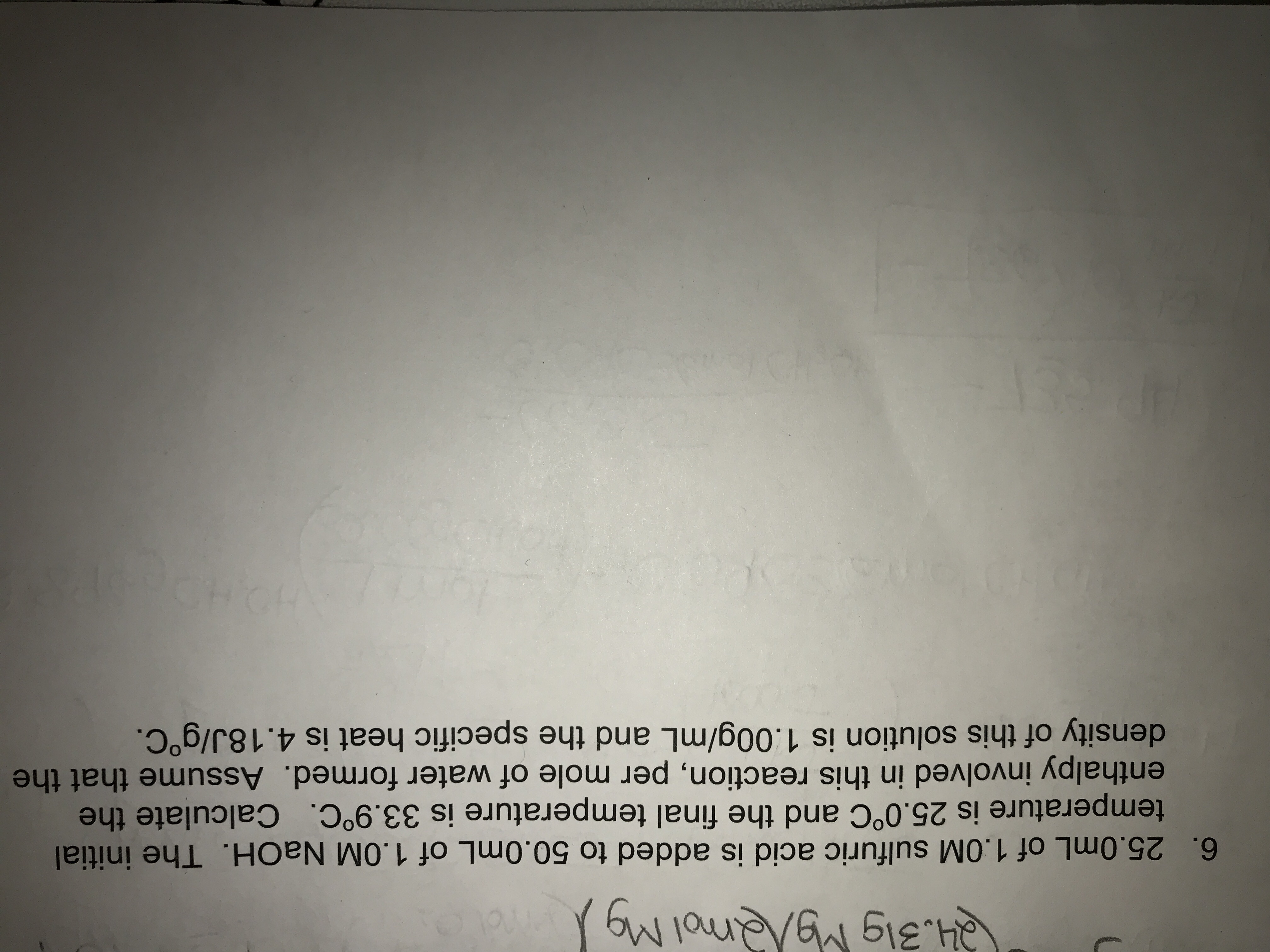6. 25.0mL of 1.0M sulfuric acid is added to 50.0mL of 1.0M NaOH. The initialtemperature is 25.0°C and the final temperature is 33.9°C. Calculate theenthalpy involved in this reaction, per mole of water formed. Assume that thedensity of this solution is 1.00g/mL and the specific heat is 4.18J/goC.

Question

Question 6help_outlineImage Transcriptionclose6. 25.0mL of 1.0M sulfuric acid is added to 50.0mL of 1.0M NaOH. The initial temperature is 25.0°C and the final temperature is 33.9°C. Calculate the enthalpy involved in this reaction, per mole of water formed. Assume that the density of this solution is 1.00g/mL and the specific heat is 4.18J/goC. fullscreen
Step 1

For the given process, the heat change may be calculated as:

Step 2

Enthalpy change for the process is heat...

Want to see the full answer?

See Solution

Want to see this answer and more?

Our solutions are written by experts, many with advanced degrees, and available 24/7

See Solution
Tagged in

Chemistry